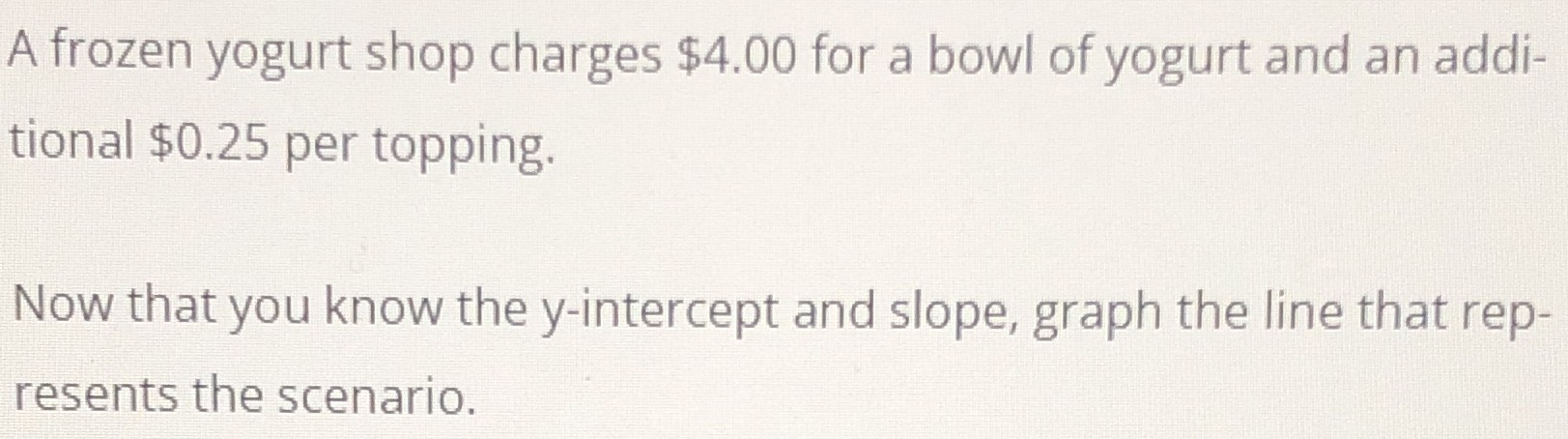### ¿Todavía tienes preguntas de matemáticas?

Pregunte a nuestros tutores expertos
Algebra
PreguntaA frozen yogurt shop charges $$\ 4.00$$ for a bowl of yogurt and an addi- tional $$\ 0.25$$ per topping. Now that you know the y-intercept and slope, graph the line that rep- resents the scenario.

Slope: $$0.25$$
$$y$$ -intercept: $$( 0,4 )$$
$$y= 0.25x+ 4$$